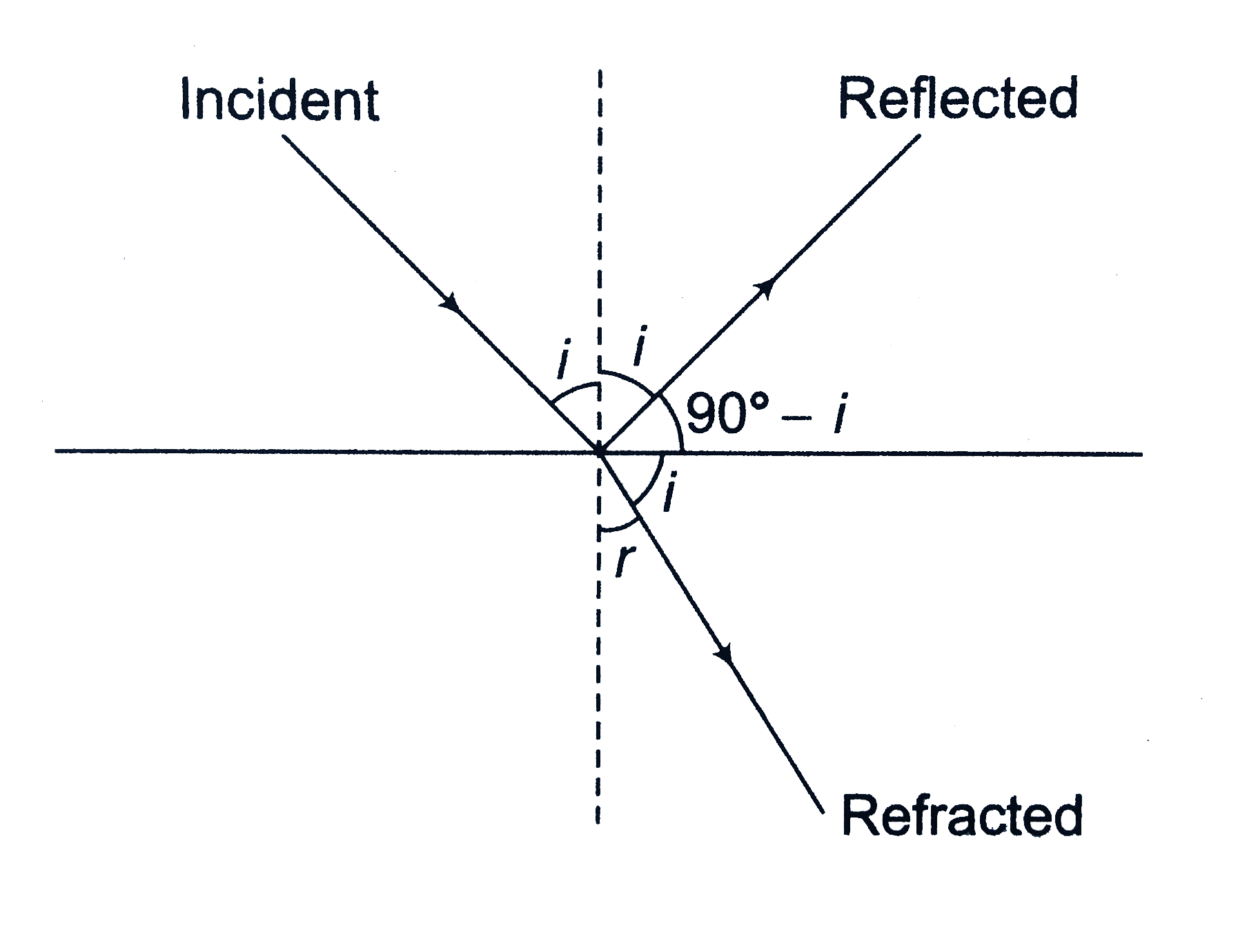Doubtnut App - Get 50 Lakh+ video solutions better than GoogleInstall Now

# A ray of light falls on a glass plate of refractive index μ=1.5. What is the angle of incidence of the ray if the angle between the reflected and refracted rays is 90∘?

Text Solution
Verified by Experts

## In the figure, r=90∘−iFrom Snell's law, 1.5=sinisinr=sinisin(90−i)=tani ∴i=tan−1(1.5)=56.3∘